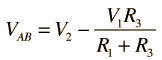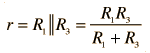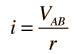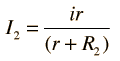# Norton: Two Loop Problem

To apply Norton's Theorem to the solution of the two loop problem, consider the current through resistor R2 below. Replacing the remainder of the network by its Norton equivalent simplifies the determination of I2 .For R1 =Ω, R2 =Ω, R3 =Ω,

 and voltages V1 = V and V2 = V,

 the open circuit voltage is= V
 since R1 and R3 form a simple voltage divider.

 The Norton resistance is= Ω.
 This gives a Norton current= A.
 Finally the calculated current is= A

Note: To avoid dealing with so many short circuits, any resistor with value zero will default to 1 when a voltage is changed. It can be changed back to a zero value if you wish to explore the effects of short circuits. Ohms and amperes are the default units, but if you put in resistor values in kilohms, then the currents will be milliamperes.

 Other approaches to two-loop circuits
Index

DC Circuits

 HyperPhysics***** Electricity and Magnetism R Nave
Go Back